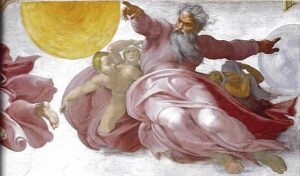# Wednesday, October 7, 2009

## Catholic Website for Young Adults

by on October 7, 2009

From my friends at iibloom:

iibloom.com HAS OFFICIALLY LAUNCHED!  Welcome to the new and exciting online community for young adults – www.iibloom.com. We would like to extend a heartfelt personal invitation for you to become part of the iibloom community.

Who? All young adults who are searching for meaning, hope, encouragement, answers, inspiration, love, and truth.

What? A state-of-the-art online community that has a mixture of mainstream, faith-based, and user-generated content as well as hot topics for discussion. The purpose of iibloom is to consistently communicate what is relevant in our lives, while also providing a welcoming and inspiring community in which we can strengthen and solidify our Christian faith through traditional Catholic teachings. A place where our voice matters!

Where? www.iibloom.com.  invite. inspire. bloom.

How can you help?
·         Invite and tell all those you know to join www.iibloom.com

Let’s see how many lives we can improve!

## Amazing Mathematics and the Love of God

by on October 7, 2009

The Universe proclaims the glory of God. Everything is not random and chance. The universe is created by an intelligent and incredible God. Notice below the Beauty of Mathematics and the Love of God!1 x 8 + 1 = 9
12 x 8 + 2 = 98
123 x 8 + 3 = 987
1234 x 8 + 4 = 9876
12345 x 8 + 5 = 98765
123456 x 8 + 6 = 987654
1234567 x 8 + 7 = 9876543
12345678 x 8 + 8 = 98765432
123456789 x 8 + 9 = 987654321

1 x 9 + 2 = 11
12 x 9 + 3 = 111
123 x 9 + 4 = 1111
1234 x 9 + 5 = 11111
12345 x 9 + 6 = 111111
123456 x 9 + 7 = 1111111
1234567 x 9 + 8 = 11111111
12345678 x 9 + 9 = 111111111
123456789 x 9 +10= 1111111111

9 x 9 + 7 = 88
98 x 9 + 6 = 888
987 x 9 + 5 = 8888
9876 x 9 + 4 = 88888
98765 x 9 + 3 = 888888
987654 x 9 + 2 = 8888888
9876543 x 9 + 1 = 88888888
98765432 x 9 + 0 = 888888888

Brilliant, isn’t it?

And look at this symmetry:

1 x 1 = 1
11 x 11 = 121
111 x 111 = 12321
1111 x 1111 = 1234321
11111 x 11111 = 123454321
111111 x 111111 = 12345654321
1111111 x 1111111 = 1234567654321
11111111 x 11111111 = 123456787654321
111111111 x 111111111 = 12345678987654321

Mind Boggling…

Now, take a look at this…

101%  —  From a strictly mathematical viewpoint:

What Equals 100%?

What does it mean to give MORE than 100%?

Ever wonder about those people who say they are giving more than 100%?

We have all been in situations where someone wants you to GIVE OVER 100%.

What equals 100% in life?

Here’s a little mathematical formula that might help answer these questions:

If:  A B C D E F G H I J K L M N O P Q R S T U V W X Y Z

Is represented as: 1 2 3 4 5 6 7 8 9 10 11 12 13 14 15 16 17 18 19 20 21 22 23 24 25 26.

Then:  H-A-R-D-W-O- R- K  equals 8+1+18+4+23+ 15+18+11 = 98%

And:  K-N-O-W-L-E- D-G-E  equals 11+14+15+23+ 12+5+4+7+ 5 = 96%

But:  A-T-T-I-T-U- D-E  equals  1+20+20+9+20+ 21+4+5 = 100%

THEN, look how far the love of God will take you:

L-O-V-E-O-F- G-O-D  equals  12+15+22+5+15+ 6+7+15+4 = 101%

Therefore, one can conclude with mathematical certainty that:

While Hard Work and Knowledge will get you close, and Attitude will get
you there, It’s the Love of God that will put you over the top!